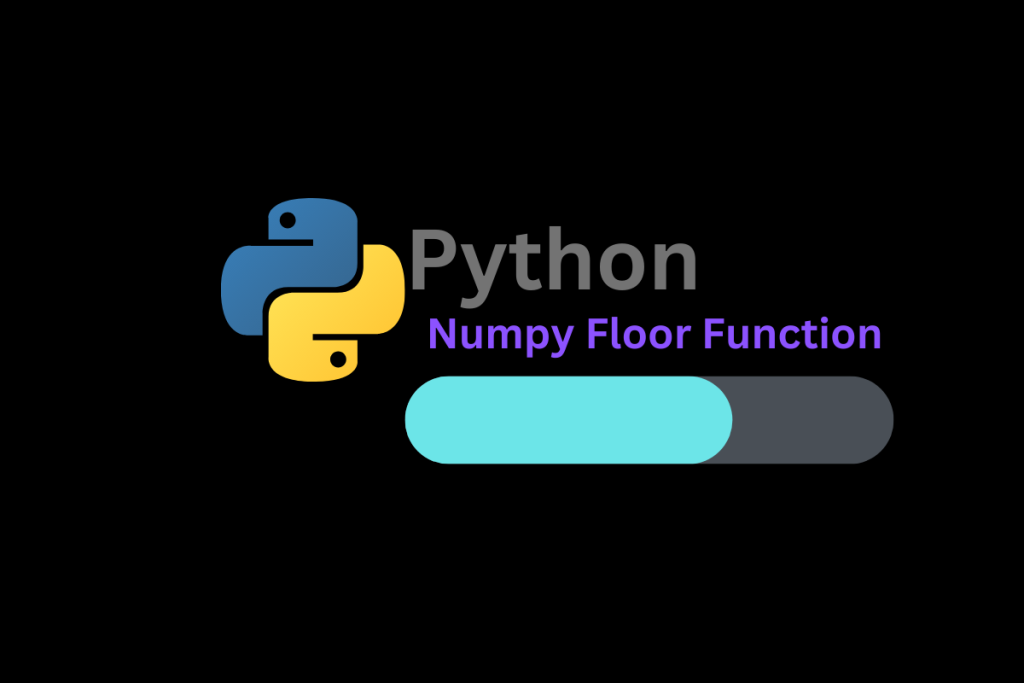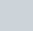# Numpy floor – Return the floor of the input, element-wiseToday we will learn how to take the floor value of an input element-wise! This means that we will be handling data element by element. We will understand how the function processes our inputs in an array or matrix form. We will also understand its implementation for all input cases. We will let the function pass all types of inputs inside and outside of its domain. Lastly, we will see its representation in a graph.

## What is NumPy?

As we are going to learn about the `numpy` floor function, Let us understand what is `NumPy`. NumPy is a Python library that provides methodologies for working with arrays. It provides some functions for working like math functions. The only difference is the syntax. Let us be a little bit theoretical.

The floor of a scalar x is the largest integer y, such that y <= x. it is dented as. Let us have a look at the examples below.

• The floor of -2.365 is -3.
• The floor of 2.365 is 2.
• The floor of any integer is the same as the number.

Now we will see how to implement the same in our code snippets. To implement this function we are using a function that is NumPy.floor() .

## Implementing the Numpy.floor() method

This function is supported by the Numpy library. To use the same we need to import its dependency which is the NumPy library. Let us see the syntax below.

```import numpy
numpy.floor(x, out=None, where=True, casting='same_kind', order='K', subok : [bool, datatype])
```

The parameters used in the above snippet function as follows:

• x: It can be a variable containing a value in Radian or it may be an array containing some value
• out: A location in which the result is to be stored. If provided, it must have a shape similar to the input X. If not provided or None, a fresh value or result is returned. It’s optional.
• where = true:  Where the condition is True, We must get our result or output for “where = False” We will not get any result. It’s optional. y default its value is True.
• casting=’same_kind’: It means only float64 to float32 value or results are allowed. The function shout cast values in this range or datatype.
• order = ‘K’: It always results in an output array in the form of k ordered. (Note: There are 4 types of orders as {‘K’, ‘C’, ‘F’, ‘A’}). It’s optional.
• subok: [bool, datatype] to make a subclass of the result or not. if True then provide a name for the subclass. It returns an array with the same shape and type as a given array. It’s also optional.

The domain for this function is the set of real numbers. The range is the set of integers. We will try our function by passing single values, arrays, and complex numbers.

### Step 1: Import Numpy

First of all, We need to import the required module which is the Numpy module.

```import numpy
```

### Step 2: Passing values to the numpy.floor() method

In this step, We will implement our function as below.

```import numpy
#Passing single value in our function
a = numpy.floor(0.366)
print(a)

#output
0.0

#passing multiple values in the form of array
import math
input = numpy.array([-2.35, 0, 0.36, 1, 5.69, math.pi, 5/2, 5%3])
b = numpy.floor(input)
print(b)

#output
[-3.  0.  0.  1.  5.  3.  2.  2.]

#passing complex number
import cmath
x=5
y=6
z=complex(x,y)
c = numpy.floor(z)
print(c)

#output
TypeError: ufunc 'floor' not supported for the input types, and the inputs could not be safely coerced to any supported types according to the casting rule ''safe''
```

You can see in the above step that, We implemented our method thrice. You can see that the floor function gives results for passing both single values, arrays, and irrational functions (`math.pi`). But in the case of passing complex numbers, It throws an error that “`ufunc 'floor' `not supported for the input types” which suggests that the input datatype is not supported by the function `NumPy.floor()`.

## Plotting numpy.floor() on a graph

In the code snippets below, We have created empty arrays x[] and y[]. Using a while loop, We have loaded input values starting from -3.99, sequentially increasing by 0.01 since 4.00 into the x[] array. After, Implementation of the floor function We loaded the respective results into the y[] array. Using both x[] and y[] arrays we have plotted our graph using `matplotlib.pyplot `library and its supportive methods.

```import matplotlib.pyplot as plt
import numpy
x=[]
y=[]
i=-3.99
while (i<4.00):
x.append(i)
y.append(numpy.floor(i))
i=i+0.01

plt.xlabel("x axis - input")
plt.ylabel("y axis - output = (numpy.floor(input))")
plt.grid(linestyle='-', linewidth=0.5,color='red')
plt.plot(x,y, linewidth=3, color = 'black')

plt.show()
```

## Conclusion

Numpy floor is a function that returns the floor of the input, element-wise. This function is useful for rounding down to the nearest integer. In this article, we have covered how to use the NumPy floor function, and how it can be applied to different data types.